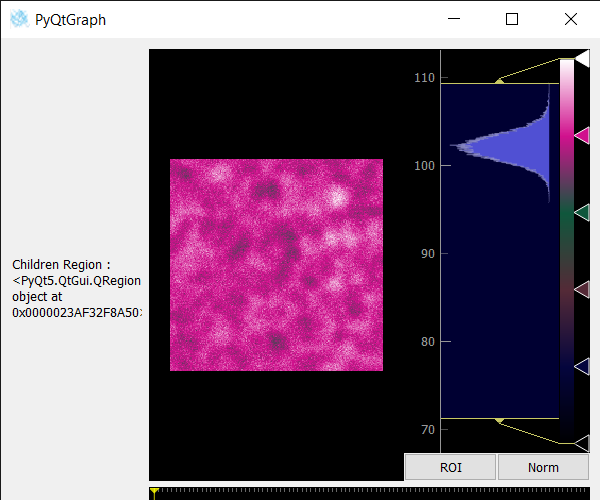# PyQtGraph – Getting Children Region of Image View

In this article, we will see how we can get children region of the image view object in PyQTGaph. The PyQtGraph is a graphics and user interface library for Python that provides functionality commonly required in designing and science applications. Its primary goals are to provide fast, interactive graphics for displaying data (plots, video, etc.), widget used for display and analysis of image data, implements many features like displaying 2D and 3D image data. For 3D data, a z-axis slider is displayed allowing the user to select which frame is displayed. Displays histogram of image data with a movable region defining the dark/light levels, editable gradient provides a color lookup table. Image view is a group of widgets like it contains a view object, push-button and scroll bars, children region is the region occupied by the children of the image view.

We can create an image view with the help of command given below:

```# creating a pyqtgraph image view object
imv = pg.ImageView()
```

In order to get children region of an Image View, we use childrenRegion() method with the image view object.

Syntax : imv.childrenRegion()
Argument : It takes no argument
Return : It returns QRegion object

Below is the program to get children region of an Image View using PyQTGaph module:

## Python3

 `# importing Qt widgets ` `from` `PyQt5.QtWidgets ``import` `*` ` `  `# importing system ` `import` `sys ` ` `  `# importing numpy as np ` `import` `numpy as np ` ` `  `# importing pyqtgraph as pg ` `import` `pyqtgraph as pg ` `from` `PyQt5.QtGui ``import` `*` `from` `PyQt5.QtCore ``import` `*` ` `  ` `  `# Image View class ` `class` `ImageView(pg.ImageView): ` ` `  `    ``# constructor which inherit original ` `    ``# ImageView ` `    ``def` `__init__(``self``, ``*``args, ``*``*``kwargs): ` `        ``pg.ImageView.__init__(``self``, ``*``args, ``*``*``kwargs) ` ` `  ` `  `class` `Window(QMainWindow): ` ` `  `    ``def` `__init__(``self``): ` `        ``super``().__init__() ` ` `  `        ``# setting title ` `        ``self``.setWindowTitle(``"PyQtGraph"``) ` ` `  `        ``# setting geometry ` `        ``self``.setGeometry(``100``, ``100``, ``600``, ``500``) ` ` `  `        ``# icon ` `        ``icon ``=` `QIcon(``"skin.png"``) ` ` `  `        ``# setting icon to the window ` `        ``self``.setWindowIcon(icon) ` ` `  `        ``# calling method ` `        ``self``.UiComponents() ` ` `  `        ``# showing all the widgets ` `        ``self``.show() ` ` `  `        ``# setting fixed size of window ` `        ``#self.setFixedSize(QSize(600, 500)) ` ` `  `    ``# method for components ` `    ``def` `UiComponents(``self``): ` ` `  `        ``# creating a widget object ` `        ``widget ``=` `QWidget() ` ` `  `        ``# creating a label ` `        ``label ``=` `QLabel(``"Geeksforgeeks Image View"``) ` ` `  `        ``# setting minimum width ` `        ``label.setMinimumWidth(``130``) ` ` `  `        ``# making label do word wrap ` `        ``label.setWordWrap(``True``) ` ` `  `        ``# setting configuration options ` `        ``pg.setConfigOptions(antialias``=``True``) ` ` `  `        ``# creating image view view object ` `        ``imv ``=` `ImageView() ` ` `  `        ``# Create random 3D data set with noisy signals ` `        ``img ``=` `pg.gaussianFilter(np.random.normal( ` `            ``size``=``(``200``, ``200``)), (``5``, ``5``)) ``*` `20` `+` `100` ` `  `        ``# setting new axis to image ` `        ``img ``=` `img[np.newaxis, :, :] ` ` `  `        ``# decay data ` `        ``decay ``=` `np.exp(``-``np.linspace(``0``, ``0.3``, ``100``))[:, np.newaxis, np.newaxis] ` ` `  `        ``# random data ` `        ``data ``=` `np.random.normal(size``=``(``100``, ``200``, ``200``)) ` `        ``data ``+``=` `img ``*` `decay ` `        ``data ``+``=` `2` ` `  `        ``# adding time-varying signal ` `        ``sig ``=` `np.zeros(data.shape[``0``]) ` `        ``sig[``30``:] ``+``=` `np.exp(``-``np.linspace(``1``, ``10``, ``70``)) ` `        ``sig[``40``:] ``+``=` `np.exp(``-``np.linspace(``1``, ``10``, ``60``)) ` `        ``sig[``70``:] ``+``=` `np.exp(``-``np.linspace(``1``, ``10``, ``30``)) ` ` `  `        ``sig ``=` `sig[:, np.newaxis, np.newaxis] ``*` `3` `        ``data[:, ``50``:``60``, ``30``:``40``] ``+``=` `sig ` ` `  `        ``# setting image to image view ` `        ``# Displaying the data and assign each frame a time value from 1.0 to 3.0 ` `        ``imv.setImage(data, xvals``=``np.linspace(``1.``, ``3.``, data.shape[``0``])) ` ` `  `        ``# Set a custom color map ` `        ``colors ``=` `[ ` `            ``(``0``, ``0``, ``0``), ` `            ``(``4``, ``5``, ``61``), ` `            ``(``84``, ``42``, ``55``), ` `            ``(``15``, ``87``, ``60``), ` `            ``(``208``, ``17``, ``141``), ` `            ``(``255``, ``255``, ``255``) ` `        ``] ` ` `  `        ``# color map ` `        ``cmap ``=` `pg.ColorMap(pos``=``np.linspace(``0.0``, ``1.0``, ``6``), color``=``colors) ` ` `  `        ``# setting color map to the image view ` `        ``imv.setColorMap(cmap) ` ` `  `        ``# Creating a grid layout ` `        ``layout ``=` `QGridLayout() ` ` `  `        ``# minimum width value of the label ` `        ``label.setFixedWidth(``130``) ` ` `  `        ``# setting this layout to the widget ` `        ``widget.setLayout(layout) ` ` `  `        ``# adding label in the layout ` `        ``layout.addWidget(label, ``1``, ``0``) ` ` `  `        ``# plot window goes on right side, spanning 3 rows ` `        ``layout.addWidget(imv, ``0``, ``1``, ``3``, ``1``) ` ` `  `        ``# setting this widget as central widget of the main widow ` `        ``self``.setCentralWidget(widget) ` ` `  `        ``# getting children region of image view ` `        ``value ``=` `imv.childrenRegion() ` ` `  `        ``# setting text to the label ` `        ``label.setText(``"Children Region : "` `+` `str``(value)) ` ` `  ` `  `# create pyqt5 app ` `App ``=` `QApplication(sys.argv) ` ` `  `# create the instance of our Window ` `window ``=` `Window() ` ` `  `# start the app ` `sys.exit(App.``exec``()) `

Output :Attention geek! Strengthen your foundations with the Python Programming Foundation Course and learn the basics.

To begin with, your interview preparations Enhance your Data Structures concepts with the Python DS Course.

My Personal Notes arrow_drop_upCheck out this Author's contributed articles.

If you like GeeksforGeeks and would like to contribute, you can also write an article using contribute.geeksforgeeks.org or mail your article to contribute@geeksforgeeks.org. See your article appearing on the GeeksforGeeks main page and help other Geeks.

Please Improve this article if you find anything incorrect by clicking on the "Improve Article" button below.

Article Tags :

Be the First to upvote.

Please write to us at contribute@geeksforgeeks.org to report any issue with the above content.